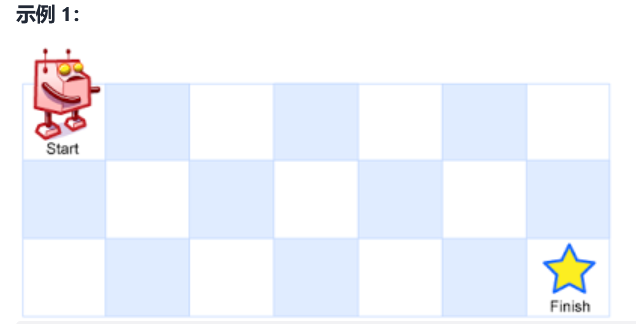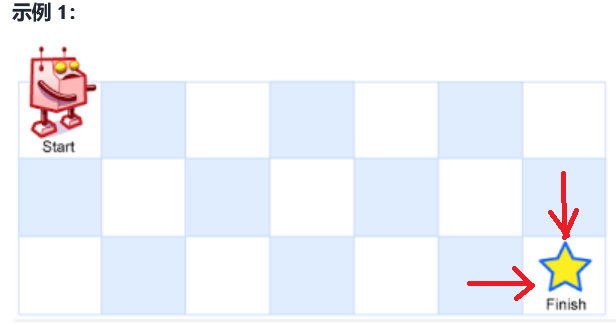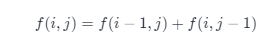IT数码 购物 网址 头条 软件 日历 阅读 图书馆
 TxT小说阅读器 ↓语音阅读,小说下载,古典文学↓ 图片批量下载器 ↓批量下载图片,美女图库↓ 图片自动播放器 ↓图片自动播放器↓ 一键清除垃圾 ↓轻轻一点,清除系统垃圾↓
 开发: C++知识库 Java知识库 JavaScript Python PHP知识库 人工智能 区块链 大数据 移动开发 嵌入式 开发工具 数据结构与算法 开发测试 游戏开发 网络协议 系统运维 教程: HTML教程 CSS教程 JavaScript教程 Go语言教程 JQuery教程 VUE教程 VUE3教程 Bootstrap教程 SQL数据库教程 C语言教程 C++教程 Java教程 Python教程 Python3教程 C#教程 数码: 电脑 笔记本 显卡 显示器 固态硬盘 硬盘 耳机 手机 iphone vivo oppo 小米 华为 单反 装机 图拉丁
 -> 数据结构与算法 -> 动态规划总结篇一 -> 正文阅读

2.动态规划使用情景一――求极值问题

1.思路分析

text1:abcd与text2:ac的最长公共子序列是ac，长度为二；
text1:abcd与text2:ace的最长公共子序列是ac,长度为二；
text1:abcde与text2:abcgef的最长公共子序列是abce,长度为四；2.代码实现

int longestCommonSubsequence(string text1, string text2) {

int n=text1.size();
int m=text2.size();
vector<vector<int>> dp(n+1,vector<int>(m+1,0));
for(int count=1;count<=n;++count){

char ch1=text1[count-1];
for(int count1=1;count1<=m;++count1){

char ch2=text2[count1-1];
if(ch1==ch2){
dp[count][count1]=dp[count-1][count1-1]+1;
}
else{
dp[count][count1]=max(dp[count-1][count1],dp[count][count1-1]);
}

}
}

return dp[n][m];
}

3.动态规划使用情景二――计数，找路径问题

1.思路分析2.代码实现

int uniquePaths(int m, int n) {

if(m==0 || n==0){
return 1;
}

vector<vector<int>> dp(m,vector<int>(n,0));
for(int count=0;count<m;++count){
dp[count]=1;
}

for(int count=0;count<n;++count){
dp[count]=1;
}

for(int count=1;count<m;++count){

for(int count1=1;count1<n;++count1){
dp[count][count1]=dp[count-1][count1]+dp[count][count1-1];
}
}

return dp[m-1][n-1];
}

4.动态规划使用情景三――求存在性问题

1.思路分析

0-1 背包最关键的就在于对于背包里的每一个元素，都有选与不选两种状态，可以选，也可以不选。因此考虑状态转移方程的时候，对每一个 dp 的值，都要从这两个状态考虑。2.代码实现

bool canPartition(vector<int>& nums) {

int n = nums.size();
if (n < 2) {
return false;
}

int sum = accumulate(nums.begin(), nums.end(), 0);
int maxNum = *max_element(nums.begin(), nums.end());
if (sum & 1) {
return false;
}

int target = sum / 2;
if (maxNum > target) {
return false;
}

vector<vector<int>> dp(n,vector<int>(target+1,0));
for(int count=0;count<n;++count){
dp[count]=true;
}

dp[nums]=true;
for(int count=1;count<n;++count){

int tmp=nums[count];
for(int count1=1;count1<=target;++count1){

if(tmp>count1){
dp[count][count1]=dp[count-1][count1];
}
else{
dp[count][count1]=dp[count-1][count1] | dp[count-1][count1-tmp];
}
}
}

return dp[n-1][target];
}

bool canPartition(vector<int>& nums) {

int n = nums.size();
if (n < 2) {
return false;
}

int sum = accumulate(nums.begin(), nums.end(), 0);
int maxNum = *max_element(nums.begin(), nums.end());
if (sum & 1) {
return false;
}

int target = sum / 2;
if (maxNum > target) {
return false;
}

vector<int> dp(target+1,0);

dp=true;
for(int count=0;count<n;++count){

int tmp=nums[count];
for(int count1=target;count1>=tmp;--count1){
dp[count1] |=dp[count1-tmp];
}
}

return dp[target];
}

5.总结

 上一篇文章      下一篇文章      查看所有文章
 加:2021-12-04 13:41:41  更:2021-12-04 13:44:18
 开发: C++知识库 Java知识库 JavaScript Python PHP知识库 人工智能 区块链 大数据 移动开发 嵌入式 开发工具 数据结构与算法 开发测试 游戏开发 网络协议 系统运维 教程: HTML教程 CSS教程 JavaScript教程 Go语言教程 JQuery教程 VUE教程 VUE3教程 Bootstrap教程 SQL数据库教程 C语言教程 C++教程 Java教程 Python教程 Python3教程 C#教程 数码: 电脑 笔记本 显卡 显示器 固态硬盘 硬盘 耳机 手机 iphone vivo oppo 小米 华为 单反 装机 图拉丁
 360图书馆 购物 三丰科技 阅读网 日历 万年历 2022年1日历 -2022/1/20 1:28:15-
 图片自动播放器 ↓图片自动播放器↓ TxT小说阅读器 ↓语音阅读,小说下载,古典文学↓ 一键清除垃圾 ↓轻轻一点,清除系统垃圾↓ 图片批量下载器 ↓批量下载图片,美女图库↓
 网站联系: qq:121756557 email:121756557@qq.com  IT数码